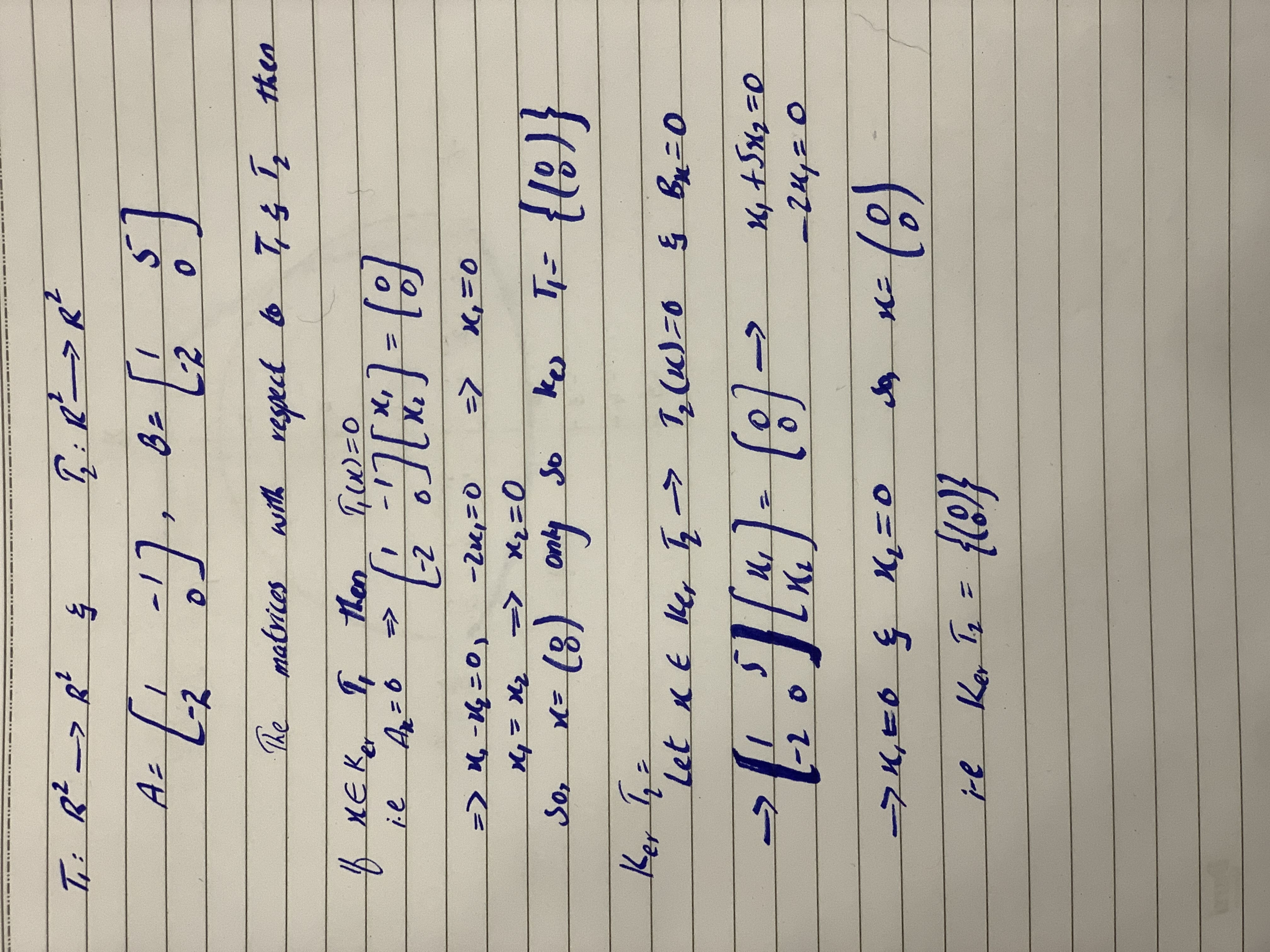# In this problem, allow T1: RR2rightarrowRR2 and T2: RR2rightarrowR2 be linear transformations. Find Ker(T_1), Ker(T_2), Ker(T_3) of the respective matricesA=[(1,-1),(-2,0)],B=[(1,5),(-2,0)]Lewis Harvey 2020-10-27 Answered

In this problem, allow be linear transformations. Find $Ker\left({T}_{1}\right),Ker\left({T}_{2}\right),Ker\left({T}_{3}\right)$ of the respective matrices
$A=\left[\left(1,-1\right),\left(-2,0\right)\right],B=\left[\left(1,5\right),\left(-2,0\right)\right]$

You can still ask an expert for help

• Questions are typically answered in as fast as 30 minutes

Solve your problem for the price of one coffee

• Math expert for every subject
• Pay only if we can solve itArnold Odonnell......................

###### Not exactly what you’re looking for?Jeffrey Jordon